# AXI4-Lite Interface Wrapper for Custom RTL in Vivado 2021.2

This project demonstrates how to take a custom RTL module and add an AXI4-Lite interface wrapper to it for use in the Vivado block design.

IntermediateFull instructions provided2 hours1,745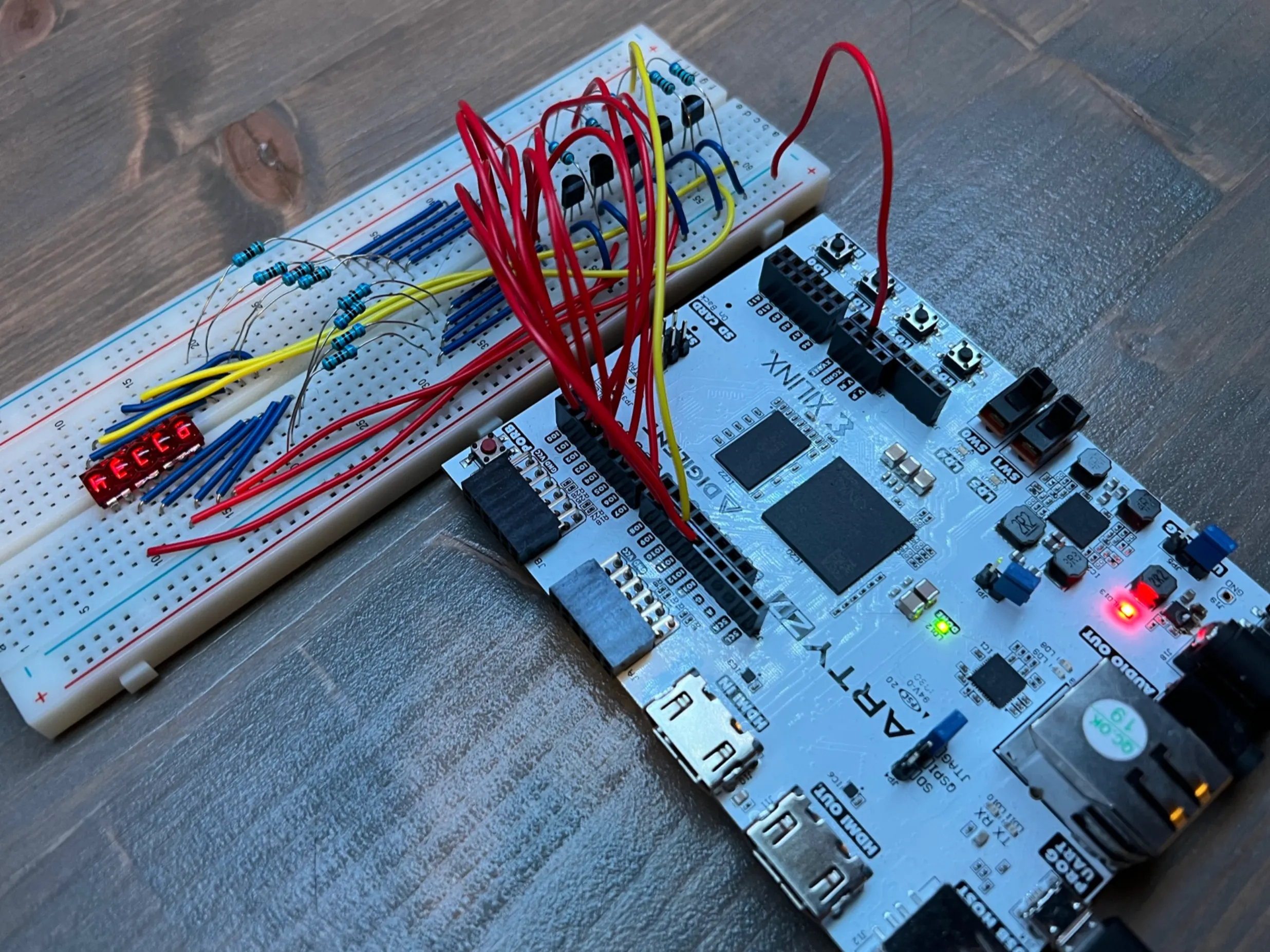## Things used in this project

### Hardware components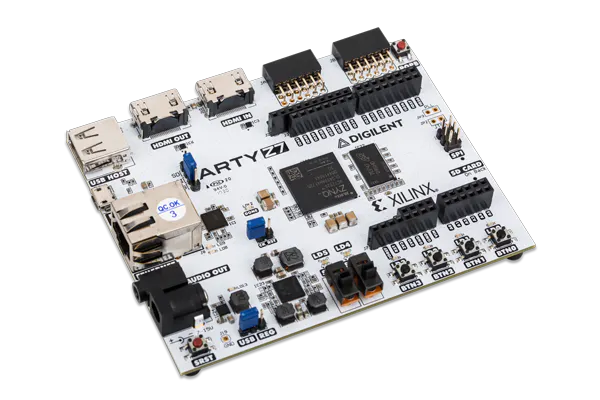Digilent Arty Z7-20
×1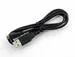USB-A to Micro-USB Cable
×1
 NPN BJT Transistor, 2N3904
×5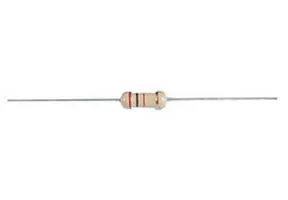Through Hole Resistor, 680 ohm
×8Through Hole Resistor, 6.8 kohm
×5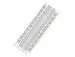×1
 HP QDSP-6061 Bubble Display (vintage)
×1

### Software apps and online servicesAMD-Xilinx Vitis Unified Software Platform

## Code

### axi_qdsp_6061_controller_v1_0.v

Verilog
````timescale 1 ns / 1 ps

module axi_qdsp_6061_controller_v1_0 #
(
// Users to add parameters here

// User parameters ends
// Do not modify the parameters beyond this line

// Parameters of Axi Slave Bus Interface S00_AXI
parameter integer C_S00_AXI_DATA_WIDTH	= 32,
)
(
// Users to add ports here
input wire logic_clk,
input wire reset,
output reg [7:0] anodeReg,
output reg [4:0] cathodeReg,
// User ports ends
// Do not modify the ports beyond this line

// Ports of Axi Slave Bus Interface S00_AXI
input wire  s00_axi_aclk,
input wire  s00_axi_aresetn,
input wire [2 : 0] s00_axi_awprot,
input wire  s00_axi_awvalid,
input wire [C_S00_AXI_DATA_WIDTH-1 : 0] s00_axi_wdata,
input wire [(C_S00_AXI_DATA_WIDTH/8)-1 : 0] s00_axi_wstrb,
input wire  s00_axi_wvalid,
output wire [1 : 0] s00_axi_bresp,
output wire  s00_axi_bvalid,
input wire [2 : 0] s00_axi_arprot,
input wire  s00_axi_arvalid,
output wire [C_S00_AXI_DATA_WIDTH-1 : 0] s00_axi_rdata,
output wire [1 : 0] s00_axi_rresp,
output wire  s00_axi_rvalid,
);
// Instantiation of Axi Bus Interface S00_AXI
wire enable;

axi_qdsp_6061_controller_v1_0_S00_AXI # (
.C_S_AXI_DATA_WIDTH(C_S00_AXI_DATA_WIDTH),
) axi_qdsp_6061_controller_v1_0_S00_AXI_inst (
.logic_enable(enable),
.S_AXI_ACLK(s00_axi_aclk),
.S_AXI_ARESETN(s00_axi_aresetn),
.S_AXI_AWPROT(s00_axi_awprot),
.S_AXI_AWVALID(s00_axi_awvalid),
.S_AXI_WDATA(s00_axi_wdata),
.S_AXI_WSTRB(s00_axi_wstrb),
.S_AXI_WVALID(s00_axi_wvalid),
.S_AXI_BRESP(s00_axi_bresp),
.S_AXI_BVALID(s00_axi_bvalid),
.S_AXI_ARPROT(s00_axi_arprot),
.S_AXI_ARVALID(s00_axi_arvalid),
.S_AXI_RDATA(s00_axi_rdata),
.S_AXI_RRESP(s00_axi_rresp),
.S_AXI_RVALID(s00_axi_rvalid),
);

parameter zero  = 8'b11111100;
parameter one   = 8'b01100000;
parameter two   = 8'b11011010;
parameter three = 8'b11110010;
parameter four  = 8'b01100110;
parameter five  = 8'b10110110;
parameter six   = 8'b10111110;
parameter seven = 8'b11100000;
parameter eight = 8'b11111110;
parameter nine  = 8'b11110110;
parameter k     = 8'b10101110;
parameter n     = 8'b00101010;
parameter i     = 8'b00001000;
parameter t     = 8'b00011110;
parameter r     = 8'b00001010;
parameter o     = 8'b00111010;
parameter c     = 8'b00011010;
parameter s     = 8'b10110110;
parameter h     = 8'b00101110;
parameter e     = 8'b10011110;
parameter l     = 8'b00011100;
parameter space = 8'b00000000;

parameter cathodeC1 = 5'b00001;
parameter cathodeC2 = 5'b00010;
parameter cathodeC3 = 5'b00100;
parameter cathodeC4 = 5'b01000;
parameter cathodeC5 = 5'b10000;

parameter msec_1 = 16'd100000; // 10ns/clk -> 0.001s/0.000000010s = 100000

reg loop_incr_flag;
reg [15:0] wait_cnt;
reg [4:0]  loop_cnt;
reg [7:0]  incr_flag_cntr;
reg [7:0]  anodeReg1, anodeReg2, anodeReg3, anodeReg4, anodeReg5;

always @ (posedge logic_clk)
begin
if (reset == 1'b0 || enable == 1'b0)
begin
wait_cnt <= 16'd0;
loop_incr_flag <= 1'b0;
cathodeReg <= cathodeC1;
end
else
begin
if (wait_cnt < msec_1)
begin
loop_incr_flag <= 1'b0;
wait_cnt <= wait_cnt + 1;
end
else
begin
wait_cnt <= 16'd0;

if (cathodeReg == cathodeC5)
begin
loop_incr_flag <= 1'b1;
cathodeReg <= cathodeC1;
end
else
begin
cathodeReg <= cathodeReg << 1;
end
end
end
end

always @ (posedge logic_clk)
begin
if (reset == 1'b0 || enable == 1'b0)
begin
loop_cnt <= 5'd0;
incr_flag_cntr <= 8'd0;
end
else
begin
if (loop_incr_flag == 1'b1)
begin
if (incr_flag_cntr == 8'd255)
begin
if (loop_cnt == 5'd15)
begin
loop_cnt <= 5'd0;
end
else
begin
loop_cnt <= loop_cnt + 1;
end
end
else
begin
loop_cnt <= loop_cnt;
end

incr_flag_cntr <= incr_flag_cntr + 1;
end
else
begin
loop_cnt <= loop_cnt;
end
end
end

always @ (posedge logic_clk)
begin
case (cathodeReg)
cathodeC1 : anodeReg[7:0] <= anodeReg1[7:0];
cathodeC2 : anodeReg[7:0] <= anodeReg2[7:0];
cathodeC3 : anodeReg[7:0] <= anodeReg3[7:0];
cathodeC4 : anodeReg[7:0] <= anodeReg4[7:0];
cathodeC5 : anodeReg[7:0] <= anodeReg5[7:0];
default   : anodeReg[7:0] <= anodeReg1[7:0];
endcase
end

always @ (posedge logic_clk)
begin
case (loop_cnt)
0 :
begin
anodeReg1[7:0] <= space;
anodeReg2[7:0] <= space;
anodeReg3[7:0] <= space;
anodeReg4[7:0] <= space;
anodeReg5[7:0] <= space;
end
1 :
begin
anodeReg1[7:0] <= space;
anodeReg2[7:0] <= space;
anodeReg3[7:0] <= space;
anodeReg4[7:0] <= space;
anodeReg5[7:0] <= h;
end
2 :
begin
anodeReg1[7:0] <= space;
anodeReg2[7:0] <= space;
anodeReg3[7:0] <= space;
anodeReg4[7:0] <= h;
anodeReg5[7:0] <= e;
end
3 :
begin
anodeReg1[7:0] <= space;
anodeReg2[7:0] <= space;
anodeReg3[7:0] <= h;
anodeReg4[7:0] <= e;
anodeReg5[7:0] <= l;
end
4 :
begin
anodeReg1[7:0] <= space;
anodeReg2[7:0] <= h;
anodeReg3[7:0] <= e;
anodeReg4[7:0] <= l;
anodeReg5[7:0] <= l;
end
5 :
begin
anodeReg1[7:0] <= h;
anodeReg2[7:0] <= e;
anodeReg3[7:0] <= l;
anodeReg4[7:0] <= l;
anodeReg5[7:0] <= o;
end
6 :
begin
anodeReg1[7:0] <= e;
anodeReg2[7:0] <= l;
anodeReg3[7:0] <= l;
anodeReg4[7:0] <= o;
anodeReg5[7:0] <= space;
end
7 :
begin
anodeReg1[7:0] <= l;
anodeReg2[7:0] <= l;
anodeReg3[7:0] <= o;
anodeReg4[7:0] <= space;
anodeReg5[7:0] <= two;
end
8 :
begin
anodeReg1[7:0] <= l;
anodeReg2[7:0] <= o;
anodeReg3[7:0] <= space;
anodeReg4[7:0] <= two;
anodeReg5[7:0] <= zero;
end
9 :
begin
anodeReg1[7:0] <= o;
anodeReg2[7:0] <= space;
anodeReg3[7:0] <= two;
anodeReg4[7:0] <= zero;
anodeReg5[7:0] <= two;
end
10 :
begin
anodeReg1[7:0] <= space;
anodeReg2[7:0] <= two;
anodeReg3[7:0] <= zero;
anodeReg4[7:0] <= two;
anodeReg5[7:0] <= two;
end
11 :
begin
anodeReg1[7:0] <= two;
anodeReg2[7:0] <= zero;
anodeReg3[7:0] <= two;
anodeReg4[7:0] <= two;
anodeReg5[7:0] <= space;
end
12 :
begin
anodeReg1[7:0] <= zero;
anodeReg2[7:0] <= two;
anodeReg3[7:0] <= two;
anodeReg4[7:0] <= space;
anodeReg5[7:0] <= space;
end
13 :
begin
anodeReg1[7:0] <= two;
anodeReg2[7:0] <= two;
anodeReg3[7:0] <= space;
anodeReg4[7:0] <= space;
anodeReg5[7:0] <= space;
end
14 :
begin
anodeReg1[7:0] <= two;
anodeReg2[7:0] <= space;
anodeReg3[7:0] <= space;
anodeReg4[7:0] <= space;
anodeReg5[7:0] <= space;
end
15 :
begin
anodeReg1[7:0] <= space;
anodeReg2[7:0] <= space;
anodeReg3[7:0] <= space;
anodeReg4[7:0] <= space;
anodeReg5[7:0] <= space;
end
default :
begin
anodeReg1[7:0] <= h;
anodeReg2[7:0] <= e;
anodeReg3[7:0] <= l;
anodeReg4[7:0] <= l;
anodeReg5[7:0] <= o;
end
endcase
end

// User logic ends

endmodule
```

### axi_qdsp_6061_controller_v1_0_S00_AXI.v

Verilog
````timescale 1 ns / 1 ps

module axi_qdsp_6061_controller_v1_0_S00_AXI #
(
// Users to add parameters here

// User parameters ends
// Do not modify the parameters beyond this line

// Width of S_AXI data bus
parameter integer C_S_AXI_DATA_WIDTH	= 32,
// Width of S_AXI address bus
)
(
// Users to add ports here
output reg logic_enable,

// User ports ends
// Do not modify the ports beyond this line

// Global Clock Signal
input wire  S_AXI_ACLK,
// Global Reset Signal. This Signal is Active LOW
input wire  S_AXI_ARESETN,
// Write address (issued by master, acceped by Slave)
// Write channel Protection type. This signal indicates the
// privilege and security level of the transaction, and whether
// the transaction is a data access or an instruction access.
input wire [2 : 0] S_AXI_AWPROT,
// Write address valid. This signal indicates that the master signaling
// valid write address and control information.
input wire  S_AXI_AWVALID,
// to accept an address and associated control signals.
// Write data (issued by master, acceped by Slave)
input wire [C_S_AXI_DATA_WIDTH-1 : 0] S_AXI_WDATA,
// Write strobes. This signal indicates which byte lanes hold
// valid data. There is one write strobe bit for each eight
// bits of the write data bus.
input wire [(C_S_AXI_DATA_WIDTH/8)-1 : 0] S_AXI_WSTRB,
// Write valid. This signal indicates that valid write
// data and strobes are available.
input wire  S_AXI_WVALID,
// Write ready. This signal indicates that the slave
// can accept the write data.
// Write response. This signal indicates the status
// of the write transaction.
output wire [1 : 0] S_AXI_BRESP,
// Write response valid. This signal indicates that the channel
// is signaling a valid write response.
output wire  S_AXI_BVALID,
// Response ready. This signal indicates that the master
// can accept a write response.
// Protection type. This signal indicates the privilege
// and security level of the transaction, and whether the
// transaction is a data access or an instruction access.
input wire [2 : 0] S_AXI_ARPROT,
input wire  S_AXI_ARVALID,
// Read data (issued by slave)
output wire [C_S_AXI_DATA_WIDTH-1 : 0] S_AXI_RDATA,
// Read response. This signal indicates the status of the
output wire [1 : 0] S_AXI_RRESP,
// Read valid. This signal indicates that the channel is
// signaling the required read data.
output wire  S_AXI_RVALID,
// accept the read data and response information.
);

// AXI4LITE signals
reg [1 : 0] 	axi_bresp;
reg  	axi_bvalid;
reg [C_S_AXI_DATA_WIDTH-1 : 0] 	axi_rdata;
reg [1 : 0] 	axi_rresp;
reg  	axi_rvalid;

// Example-specific design signals
// local parameter for addressing 32 bit / 64 bit C_S_AXI_DATA_WIDTH
// ADDR_LSB = 2 for 32 bits (n downto 2)
// ADDR_LSB = 3 for 64 bits (n downto 3)
localparam integer ADDR_LSB = (C_S_AXI_DATA_WIDTH/32) + 1;
//----------------------------------------------
//-- Signals for user logic register space example
//------------------------------------------------
//-- Number of Slave Registers 4
reg [C_S_AXI_DATA_WIDTH-1:0]	slv_reg0;
reg [C_S_AXI_DATA_WIDTH-1:0]	slv_reg1;
reg [C_S_AXI_DATA_WIDTH-1:0]	slv_reg2;
reg [C_S_AXI_DATA_WIDTH-1:0]	slv_reg3;
wire	 slv_reg_rden;
wire	 slv_reg_wren;
reg [C_S_AXI_DATA_WIDTH-1:0]	 reg_data_out;
integer	 byte_index;
reg	 aw_en;

// I/O Connections assignments

assign S_AXI_BRESP	= axi_bresp;
assign S_AXI_BVALID	= axi_bvalid;
assign S_AXI_RDATA	= axi_rdata;
assign S_AXI_RRESP	= axi_rresp;
assign S_AXI_RVALID	= axi_rvalid;
// axi_awready is asserted for one S_AXI_ACLK clock cycle when both
// S_AXI_AWVALID and S_AXI_WVALID are asserted. axi_awready is
// de-asserted when reset is low.

always @( posedge S_AXI_ACLK )
begin
if ( S_AXI_ARESETN == 1'b0 )
begin
aw_en <= 1'b1;
end
else
begin
if (~axi_awready && S_AXI_AWVALID && S_AXI_WVALID && aw_en)
begin
// there is a valid write address and write data
// on the write address and data bus. This design
// expects no outstanding transactions.
aw_en <= 1'b0;
end
begin
aw_en <= 1'b1;
end
else
begin
end
end
end

// This process is used to latch the address when both
// S_AXI_AWVALID and S_AXI_WVALID are valid.

always @( posedge S_AXI_ACLK )
begin
if ( S_AXI_ARESETN == 1'b0 )
begin
end
else
begin
if (~axi_awready && S_AXI_AWVALID && S_AXI_WVALID && aw_en)
begin
end
end
end

// axi_wready is asserted for one S_AXI_ACLK clock cycle when both
// S_AXI_AWVALID and S_AXI_WVALID are asserted. axi_wready is
// de-asserted when reset is low.

always @( posedge S_AXI_ACLK )
begin
if ( S_AXI_ARESETN == 1'b0 )
begin
end
else
begin
if (~axi_wready && S_AXI_WVALID && S_AXI_AWVALID && aw_en )
begin
// slave is ready to accept write data when
// there is a valid write address and write data
// on the write address and data bus. This design
// expects no outstanding transactions.
end
else
begin
end
end
end

// Implement memory mapped register select and write logic generation
// The write data is accepted and written to memory mapped registers when
// axi_awready, S_AXI_WVALID, axi_wready and S_AXI_WVALID are asserted. Write strobes are used to
// select byte enables of slave registers while writing.
// These registers are cleared when reset (active low) is applied.
// Slave register write enable is asserted when valid address and data are available
// and the slave is ready to accept the write address and write data.

always @( posedge S_AXI_ACLK )
begin
if ( S_AXI_ARESETN == 1'b0 )
begin
slv_reg0 <= 0;
slv_reg1 <= 0;
slv_reg2 <= 0;
slv_reg3 <= 0;
end
else begin
if (slv_reg_wren)
begin
2'h0:
for ( byte_index = 0; byte_index <= (C_S_AXI_DATA_WIDTH/8)-1; byte_index = byte_index+1 )
if ( S_AXI_WSTRB[byte_index] == 1 ) begin
// Respective byte enables are asserted as per write strobes
// Slave register 0
slv_reg0[(byte_index*8) +: 8] <= S_AXI_WDATA[(byte_index*8) +: 8];
end
2'h1:
for ( byte_index = 0; byte_index <= (C_S_AXI_DATA_WIDTH/8)-1; byte_index = byte_index+1 )
if ( S_AXI_WSTRB[byte_index] == 1 ) begin
// Respective byte enables are asserted as per write strobes
// Slave register 1
slv_reg1[(byte_index*8) +: 8] <= S_AXI_WDATA[(byte_index*8) +: 8];
end
2'h2:
for ( byte_index = 0; byte_index <= (C_S_AXI_DATA_WIDTH/8)-1; byte_index = byte_index+1 )
if ( S_AXI_WSTRB[byte_index] == 1 ) begin
// Respective byte enables are asserted as per write strobes
// Slave register 2
slv_reg2[(byte_index*8) +: 8] <= S_AXI_WDATA[(byte_index*8) +: 8];
end
2'h3:
for ( byte_index = 0; byte_index <= (C_S_AXI_DATA_WIDTH/8)-1; byte_index = byte_index+1 )
if ( S_AXI_WSTRB[byte_index] == 1 ) begin
// Respective byte enables are asserted as per write strobes
// Slave register 3
slv_reg3[(byte_index*8) +: 8] <= S_AXI_WDATA[(byte_index*8) +: 8];
end
default : begin
slv_reg0 <= slv_reg0;
slv_reg1 <= slv_reg1;
slv_reg2 <= slv_reg2;
slv_reg3 <= slv_reg3;
end
endcase
end
end
end

// Implement write response logic generation
// The write response and response valid signals are asserted by the slave
// This marks the acceptance of address and indicates the status of
// write transaction.

always @( posedge S_AXI_ACLK )
begin
if ( S_AXI_ARESETN == 1'b0 )
begin
axi_bvalid  <= 0;
axi_bresp   <= 2'b0;
end
else
begin
begin
// indicates a valid write response is available
axi_bvalid <= 1'b1;
axi_bresp  <= 2'b0; // 'OKAY' response
end                   // work error responses in future
else
begin
//check if bready is asserted while bvalid is high)
//(there is a possibility that bready is always asserted high)
begin
axi_bvalid <= 1'b0;
end
end
end
end

// axi_arready is asserted for one S_AXI_ACLK clock cycle when
// S_AXI_ARVALID is asserted. axi_awready is
// de-asserted when reset (active low) is asserted.
// asserted. axi_araddr is reset to zero on reset assertion.

always @( posedge S_AXI_ACLK )
begin
if ( S_AXI_ARESETN == 1'b0 )
begin
end
else
begin
begin
end
else
begin
end
end
end

// Implement axi_arvalid generation
// axi_rvalid is asserted for one S_AXI_ACLK clock cycle when both
// S_AXI_ARVALID and axi_arready are asserted. The slave registers
// data are available on the axi_rdata bus at this instance. The
// assertion of axi_rvalid marks the validity of read data on the
// bus and axi_rresp indicates the status of read transaction.axi_rvalid
// is deasserted on reset (active low). axi_rresp and axi_rdata are
// cleared to zero on reset (active low).
always @( posedge S_AXI_ACLK )
begin
if ( S_AXI_ARESETN == 1'b0 )
begin
axi_rvalid <= 0;
axi_rresp  <= 0;
end
else
begin
if (axi_arready && S_AXI_ARVALID && ~axi_rvalid)
begin
axi_rvalid <= 1'b1;
axi_rresp  <= 2'b0; // 'OKAY' response
end
begin
// Read data is accepted by the master
axi_rvalid <= 1'b0;
end
end
end

// Implement memory mapped register select and read logic generation
// Slave register read enable is asserted when valid address is available
assign slv_reg_rden = axi_arready & S_AXI_ARVALID & ~axi_rvalid;
always @(*)
begin
2'h0   : reg_data_out <= slv_reg0;
2'h1   : reg_data_out <= slv_reg1;
2'h2   : reg_data_out <= slv_reg2;
2'h3   : reg_data_out <= slv_reg3;
default : reg_data_out <= 0;
endcase
end

// Output register or memory read data
always @( posedge S_AXI_ACLK )
begin
if ( S_AXI_ARESETN == 1'b0 )
begin
axi_rdata  <= 0;
end
else
begin
if (slv_reg_rden)
begin
axi_rdata <= reg_data_out;     // register read data
end
end
end

always @ (posedge S_AXI_ACLK)
begin
if (S_AXI_ARESETN == 1'b0)
begin
logic_enable <= 1'b0;
end
else
begin
if (slv_reg0 == 32'hffffffff)
begin
logic_enable <= 1'b1;
end
else
begin
logic_enable <= logic_enable;
end
end
end

// User logic ends

endmodule
```

### axi_qdsp_6061_controller.c

C/C++
```/***************************** Include Files *******************************/
#include "axi_qdsp_6061_controller.h"
#include "xparameters.h"
#include "stdio.h"
#include "xil_io.h"

#define slave_reg0    AXI_QDSP_6061_CONTROLLER_S00_AXI_SLV_REG0_OFFSET
#define slave_reg1    AXI_QDSP_6061_CONTROLLER_S00_AXI_SLV_REG1_OFFSET
#define slave_reg2    AXI_QDSP_6061_CONTROLLER_S00_AXI_SLV_REG2_OFFSET
#define slave_reg3    AXI_QDSP_6061_CONTROLLER_S00_AXI_SLV_REG3_OFFSET
/************************** Function Definitions ***************************/

return XST_SUCCESS;

}

u32 data;

}

return *data;

}
```

### axi_qdsp_6061_controller.h

```#ifndef AXI_QDSP_6061_CONTROLLER_H
#define AXI_QDSP_6061_CONTROLLER_H

/****************** Include Files ********************/
#include "xil_types.h"
#include "xstatus.h"

#define AXI_QDSP_6061_CONTROLLER_S00_AXI_SLV_REG0_OFFSET 0
#define AXI_QDSP_6061_CONTROLLER_S00_AXI_SLV_REG1_OFFSET 4
#define AXI_QDSP_6061_CONTROLLER_S00_AXI_SLV_REG2_OFFSET 8
#define AXI_QDSP_6061_CONTROLLER_S00_AXI_SLV_REG3_OFFSET 12

/**************************** Type Definitions *****************************/
/**
*
* Write a value to a AXI_QDSP_6061_CONTROLLER register. A 32 bit write is performed.
* If the component is implemented in a smaller width, only the least
* significant data is written.
*
* @param   RegOffset is the register offset from the base to write to.
* @param   Data is the data written to the register.
*
* @return  None.
*
* @note
* C-style signature:
* 	void AXI_QDSP_6061_CONTROLLER_mWriteReg(u32 BaseAddress, unsigned RegOffset, u32 Data)
*
*/

/**
*
* Read a value from a AXI_QDSP_6061_CONTROLLER register. A 32 bit read is performed.
* If the component is implemented in a smaller width, only the least
* significant data is read from the register. The most significant data
* will be read as 0.
*
* @param   RegOffset is the register offset from the base to write to.
*
* @return  Data is the data from the register.
*
* @note
* C-style signature:
*
*/

/************************** Function Prototypes ****************************/
/**
*
* Run a self-test on the driver/device. Note this may be a destructive test if
* resets of the device are performed.
*
* If the hardware system is not built correctly, this function may never
*
* @param   baseaddr_p is the base address of the AXI_QDSP_6061_CONTROLLER instance to be worked on.
*
* @return
*
*    - XST_SUCCESS   if all self-test code passed
*    - XST_FAILURE   if any self-test code failed
*
* @note    Caching must be turned off for this function to work.
* @note    Self test may fail if data memory and device are not on the same bus.
*
*/

#endif // AXI_QDSP_6061_CONTROLLER_H
```

### main.c

C/C++
```/******************************************************************************
*
*
* Permission is hereby granted, free of charge, to any person obtaining a copy
* of this software and associated documentation files (the "Software"), to deal
* in the Software without restriction, including without limitation the rights
* to use, copy, modify, merge, publish, distribute, sublicense, and/or sell
* copies of the Software, and to permit persons to whom the Software is
* furnished to do so, subject to the following conditions:
*
* The above copyright notice and this permission notice shall be included in
* all copies or substantial portions of the Software.
*
* Use of the Software is limited solely to applications:
* (a) running on a Xilinx device, or
* (b) that interact with a Xilinx device through a bus or interconnect.
*
* THE SOFTWARE IS PROVIDED "AS IS", WITHOUT WARRANTY OF ANY KIND, EXPRESS OR
* IMPLIED, INCLUDING BUT NOT LIMITED TO THE WARRANTIES OF MERCHANTABILITY,
* FITNESS FOR A PARTICULAR PURPOSE AND NONINFRINGEMENT. IN NO EVENT SHALL
* XILINX  BE LIABLE FOR ANY CLAIM, DAMAGES OR OTHER LIABILITY,
* WHETHER IN AN ACTION OF CONTRACT, TORT OR OTHERWISE, ARISING FROM, OUT OF
* OR IN CONNECTION WITH THE SOFTWARE OR THE USE OR OTHER DEALINGS IN THE
* SOFTWARE.
*
* Except as contained in this notice, the name of the Xilinx shall not be used
* in advertising or otherwise to promote the sale, use or other dealings in
* this Software without prior written authorization from Xilinx.
*
******************************************************************************/

/*
* helloworld.c: simple test application
*
* This application configures UART 16550 to baud rate 9600.
* PS7 UART (Zynq) is not initialized by this application, since
* bootrom/bsp configures it to baud rate 115200
*
* ------------------------------------------------
* | UART TYPE   BAUD RATE                        |
* ------------------------------------------------
*   uartns550   9600
*   uartlite    Configurable only in HW design
*   ps7_uart    115200 (configured by bootrom/bsp)
*/

#include <stdio.h>
#include <string.h>
#include <stdlib.h>
#include <unistd.h>

#include "stdio.h"
#include "xgpio.h"
#include "xil_io.h"
#include "xstatus.h"
#include "platform.h"
#include "xil_types.h"
#include "xil_printf.h"
#include "xil_assert.h"
#include "xparameters.h"
#include "xil_exception.h"
#include "axi_qdsp_6061_controller.h"

#define LEDs_DeviceID           XPAR_AXI_GPIO_LEDS_DEVICE_ID
#define QDSP6061_DeviceID       XPAR_AXI_QDSP_6061_CONTRO_0_DEVICE_ID

int status;

int main()
{
init_platform();

print("Hello World!\n\r");

if(status != XST_SUCCESS)
{
xil_printf("Failed to write to registers of QDSP-6061 Interface.\n\r");
return XST_FAILURE;
}

print("Successfully ran Hello World application");
cleanup_platform();
return 0;
}
```

## Credits

### Whitney Knitter

28 projects • 943 followers
Working as a full-time SDR/FPGA engineer, but making time for the fun projects at home.